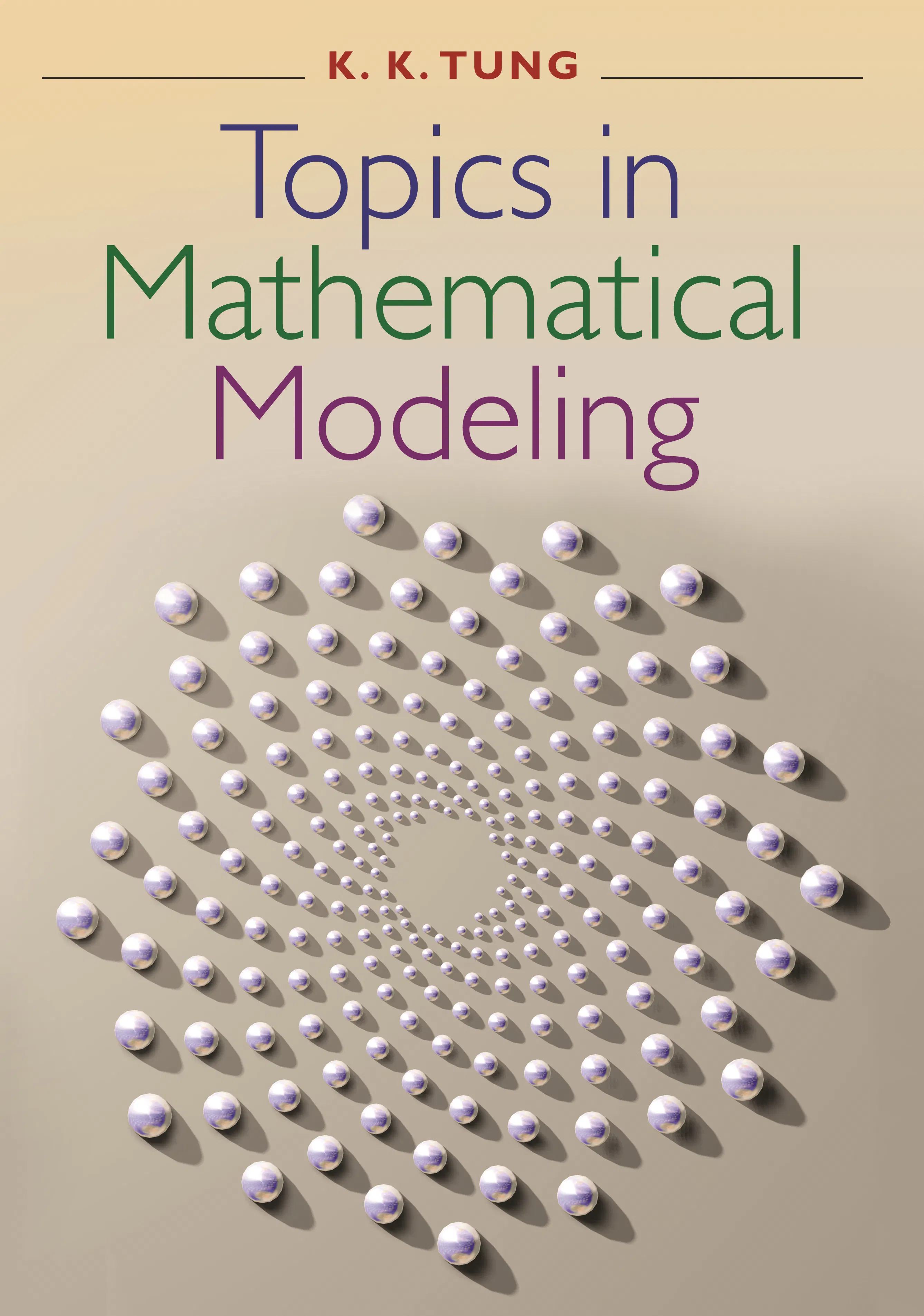#### Topics in Mathematical Modeling

K. K. Tung

Audiobooks and ebooks purchased from this site must be accessed on the Princeton University Press app.

# Topics in Mathematical Modeling## Hardcover

Price:
\$95.00/£80.00
ISBN:
Published:
Apr 15, 2007
2007
Pages:
336
Size:
7 x 10 in.
Illus:
31 halftones. 52 line illus. 5 tables.

Topics in Mathematical Modeling is an introductory textbook on mathematical modeling. The book teaches how simple mathematics can help formulate and solve real problems of current research interest in a wide range of fields, including biology, ecology, computer science, geophysics, engineering, and the social sciences. Yet the prerequisites are minimal: calculus and elementary differential equations. Among the many topics addressed are HIV; plant phyllotaxis; global warming; the World Wide Web; plant and animal vascular networks; social networks; chaos and fractals; marriage and divorce; and El Niño. Traditional modeling topics such as predator-prey interaction, harvesting, and wars of attrition are also included. Most chapters begin with the history of a problem, follow with a demonstration of how it can be modeled using various mathematical tools, and close with a discussion of its remaining unsolved aspects.

Designed for a one-semester course, the book progresses from problems that can be solved with relatively simple mathematics to ones that require more sophisticated methods. The math techniques are taught as needed to solve the problem being addressed, and each chapter is designed to be largely independent to give teachers flexibility.

The book, which can be used as an overview and introduction to applied mathematics, is particularly suitable for sophomore, junior, and senior students in math, science, and engineering.